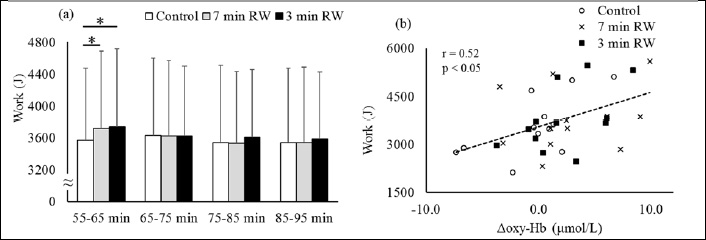Figure 2. The mean work at each measurement point among three trials (a) and correlation between the mean work at 55-65 min and the mean of the changes in oxygenated hemoglobin concentration during low-intensity exercise in 55-65 min (b). Control: 15 min of seated rest trial, 7 min RW: 7 min of the cycling at 70% of HRmax trial, 3 min RW: 3 min of the cycling at 70% of HRmax trial. (n=13, mean ± SD). Means were compared by using repeated measures two-factor analysis of variance followed by a Bonferroni multiple comparisons test (Figure 2a). The mean work: a main effect of trial; p > 0.05, a main effect of time; p < 0.05, interaction; p < 0.05. * Significantly different from the control trial (p < 0.05). The correlation coefficients were determined by using Pearson’s product-moment test (Figure 2b).